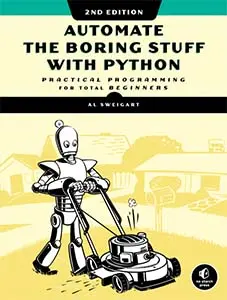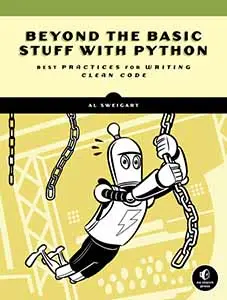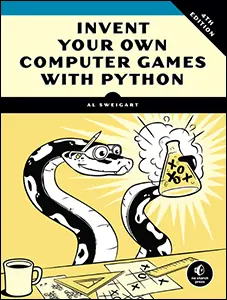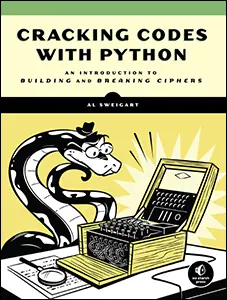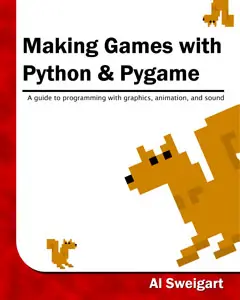# The Invent with Python Blog

Writings from the author of Automate the Boring Stuff.

Wed 09 March 2022

# Make Fractals in Python Turtle with the FractalArtMaker Module

Posted by Al Sweigart in python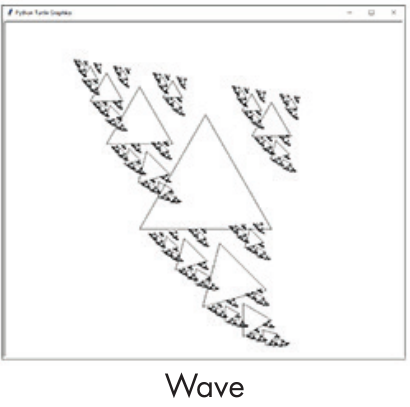FractalArtMaker is a module for creating fractal art in Python's `turtle` module. You can install the module by running `pip3 install FractalArtMaker`

This module is explored in the book "The Recursive Book of Recursion" by Al Sweigart from No Starch Press. This blog post will show you how to make fractals like the following: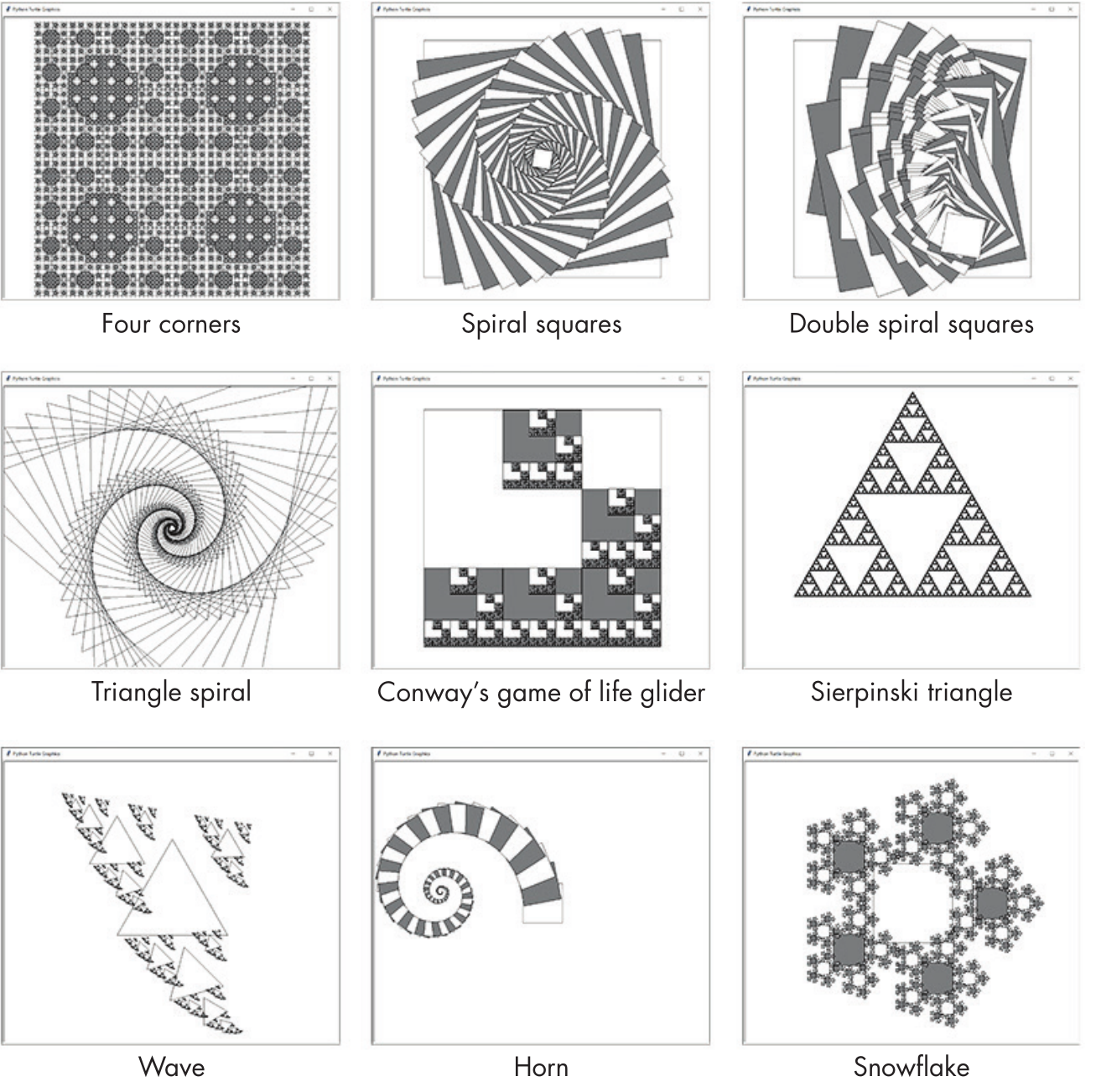You can purchase this book directly from the publisher at https://nostarch.com/recursive-book-recursion or read it online at https://inventwithpython.com/recursion/

## Quickstart

To view some example fractals, run the following from the interactive shell:

```>>> import fractalartmaker
>>> fractalartmaker.fast()  # draw the fractals quickly
>>> fractalartmaker.example(1)  # pass 1 to 9
```

## Making Fractals

The Fractal Art Maker's algorithm has two major components: a shape-drawing function and the recursive `drawFractal()` function.

The shape-drawing function draws a basic shape. The Fractal Art Maker program comes with the two shape-drawing functions, `fractalartmaker.drawFilledSquare()` and `fractalartmaker.drawTriangleOutline()`, but you can also create your own. We pass a shape-drawing function to the `fractalartmaker.drawFractal()` function as an argument.

The `fractalartmaker.drawFractal()` function also has a parameter indicating changes to the size, position, and angle of the shapes between recursive calls to `fractalartmaker.drawFractal()`.

The `fractalartmaker.drawFractal()` function uses a shape-drawing function passed to it to draw the individual parts of the fractal. This is usually a simple shape, such as a square or triangle. The beautiful complexity of the fractals emerges from `fractalartmaker.drawFractal()` recursively calling this function for each individual component of the whole fractal.

Here's the two shape-drawing functions that come in the `fractalartmaker` module:

```def drawFilledSquare(size, depth):
size = int(size)

# Move to the top-right corner before drawing:
turtle.penup()
turtle.forward(size // 2)
turtle.left(90)
turtle.forward(size // 2)
turtle.left(180)
turtle.pendown()

# Alternate between white and gray (with black border):
if depth % 2 == 0:
turtle.pencolor('black')
turtle.fillcolor('white')
else:
turtle.pencolor('black')
turtle.fillcolor('gray')

# Draw a square:
turtle.begin_fill()
for i in range(4):  # Draw four lines.
turtle.forward(size)
turtle.right(90)
turtle.end_fill()

def drawTriangleOutline(size, depth):
size = int(size)

# Move the turtle to the top of the equilateral triangle:
height = size * math.sqrt(3) / 2
turtle.penup()
turtle.left(90)  # Turn to face upwards.
turtle.forward(height * (2/3))  # Move to the top corner.
turtle.right(150)  # Turn to face the bottom-right corner.
turtle.pendown()

# Draw the three sides of the triangle:
for i in range(3):
turtle.forward(size)
turtle.right(120)
```

The shape-drawing functions for the Fractal Art Maker have two parameters: `size` and `depth`. The size parameter is the length of the sides of the square or triangle it draws. The shape-drawing functions should always use arguments to `turtle.forward()` that are based on `size` so that the lengths will be proportionate to size at each level of recursion. Avoid code like `turtle.forward(100)` or `turtle.forward(200)`; instead, use code that is based on the `size` parameter, like `turtle.forward(size)` or `turtle.forward(size * 2)`. In Python's `turtle` module, `turtle.forward(1)` moves the turtle by one unit, which is not necessarily the same as one pixel.

The shape-drawing functions' second parameter is the recursive depth of `fractalartmaker.drawFractal()`. Your shape-drawing function can ignore this argument, but using it can cause interesting variations to the basic shape. For example, the `fractalartmaker.drawFilledSquare()` shape-drawing function uses depth to alternate between drawing white squares and gray squares. Keep this in mind if you'd like to create your own shape-drawing functions for the Fractal Art Maker program, as they must accept a `size` and `depth` argument.

The `fractalartmaker.drawFractal()` function has three required parameters and one optional one: `shapeDrawFunction`, `size`, `specs`, and optionally `maxDepth`. The `shapeDrawFunction` parameter expects a function, like `fractalartmaker.drawFilledSquare` or `fractalartmaker.drawTriangleOutline`. The `size` parameter expects the starting size passed to the drawing function. Often, a value between `100` and `500` is a good starting size, though this depends on the code in your shape-drawing function, and finding the right value may require experimentation.

The `specs` parameter expects a list of dictionaries that specify how the recursive shapes should change their size, position, and angle as `fractalartmaker.drawFractal()` recursively calls itself. These specifications are described later in this section. To prevent `fractalartmaker.drawFractal()` from recursing until it causes a stack overflow, the `maxDepth` parameter holds the number of times `fractalartmaker.drawFractal()` should recursively call itself. By default, `maxDepth` has a value of `8`, but you can provide a different value if you want more recursive shapes or fewer.

The recursive calls to `fractalartmaker.drawFractal()` are based on the specification in the `specs` list's dictionaries. For each dictionary, `fractalartmaker.drawFractal()` makes one recursive call to `fractalartmaker.drawFractal()`. If specs is a list with one dictionary, every call to `fractalartmaker.drawFractal()` results in only one recursive call to `fractalartmaker.drawFractal()`. If specs is a list with three dictionaries, every call to `fractalartmaker.drawFractal()` results in three recursive calls to `fractalartmaker.drawFractal()`.

The dictionaries in the specs parameter provide specifications for each recursive call. Each of these dictionaries has the keys `sizeChange`, `xChange`, `yChange`, and `angleChange`. These dictate how the size of the fractal, the position of the turtle, and the heading of the turtle change for a recursive `fractalartmaker.drawFractal()` call.

* `sizeChange` (default is `1.0`) - The next recursive shape's size value is the current size multiplied by this value. * `xChange` (default is `0.0`) - The next recursive shape's x-coordinate is the current x-coordinate plus the current size multiplied by this value. * `yChange` (default is `0.0`) - The next recursive shape's y-coordinate is the current y-coordinate plus the current size multiplied by this value. * `angleChange` (default is `0.0`) - The next recursive shape's starting angle is the current starting angle plus this value.

Let's take a look at the specification dictionary for the Four Corners fractal, which is drawn when you call `fractalartmaker.example(1)`. The call to `fractalartmaker.drawFractal()` for the Four Corners fractal passes the following list of dictionaries for the `specs` parameter:

```fractalartmaker.drawFractal(fractalartmaker.drawFilledSquare, 350,
[{'sizeChange': 0.5, 'xChange': -0.5, 'yChange': 0.5},
{'sizeChange': 0.5, 'xChange': 0.5, 'yChange': 0.5},
{'sizeChange': 0.5, 'xChange': -0.5, 'yChange': -0.5},
{'sizeChange': 0.5, 'xChange': 0.5, 'yChange': -0.5}], 5)
```

The `specs` list has four dictionaries, so each call to `drawFractal()` that draws a square will, in turn, recursively call `drawFractal()` four more times to draw four more squares.

To determine the size of the next square to be drawn, the value for the `sizeChange` key is multiplied by the current size parameter. The first dictionary in the specs list has a `sizeChange` value of `0.5`, which makes the next recursive call have a size argument of `350 * 0.5`, or `175` units. This makes the next square half the size of the previous square. A `sizeChange` value of `2.0` would, for example, double the size of the next square. If the dictionary has no `sizeChange` key, the value defaults to `1.0` for no change to the size.

If you look at the three other dictionaries in the `specs` list, you'll notice they all have a `sizeChange` value of `0.5`. The difference between them is that their `xChange` and `yChange` values place them in the other three corners of the current square. As a result, the next four squares are drawn centered on the four corners of the current square.

The dictionaries in the `specs` list for this example don't have an `angleChange` value, so this value defaults to `0.0` degrees. A positive `angleChange` value indicates a counterclockwise rotation, while a negative value indicates a clockwise rotation.

Take a look at the code in the module's `example()` function for more examples.

The `fractalartmaker` module also has a `fractalartmaker.fast()` function you can call to make the fractals draw quickly, and a `fractalartmaker.clear()` to clear the turtle drawing window.

Let's examine the code for each of the 9 example fractals that come with the module.

## Example 1 - Four Corners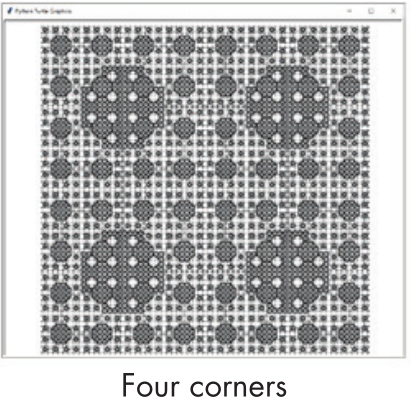The first fractal is Four Corners, which begins as a large square. As the function calls itself, the fractal's specifications cause four smaller squares to be drawn in the four corners of the square:

```# Four Corners:
drawFractal(drawFilledSquare, 350,
[{'sizeChange': 0.5, 'xChange': -0.5, 'yChange': 0.5},
{'sizeChange': 0.5, 'xChange': 0.5, 'yChange': 0.5},
{'sizeChange': 0.5, 'xChange': -0.5, 'yChange': -0.5},
{'sizeChange': 0.5, 'xChange': 0.5, 'yChange': -0.5}], 5)
```

The call to `drawFractal()` here limits the maximum depth to `5`, as any more tends to make the fractal so dense that the fine detail becomes hard to see.

## Example 2 - Spiral Squares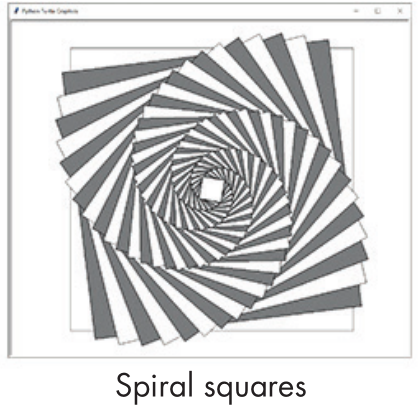The Spiral Squares fractal also starts as a large square, but it creates just one new square on each recursive call:

```# Spiral Squares:
drawFractal(drawFilledSquare, 600, [{'sizeChange': 0.95, 'angleChange': 7}], 50)
```

This square is slightly smaller and rotated by 7 degrees. The centers of all the squares are unchanged, so there's no need to add `xChange` and `yChange` keys `to` the specification. The default maximum depth of `8` is `too` small to get an interesting fractal, so we increase it to `50` to `produce` a hypnotic spiral pattern.

## Example 3 - Double Spiral SquaresThe Double Spiral Squares fractal is similar to Spiral Squares, except each square creates two smaller squares. This creates an interesting fan effect, as the second square is drawn later and tends to cover up previously drawn squares:

```# Double Spiral Squares:
drawFractal(drawFilledSquare, 600,
[{'sizeChange': 0.8, 'yChange': 0.1, 'angleChange': -10},
{'sizeChange': 0.8, 'yChange': -0.1, 'angleChange': 10}])
```

The squares are created slightly higher or lower than their previous square and rotated either `10` or `-10` degrees.

## Example 4 - Triangle Spiral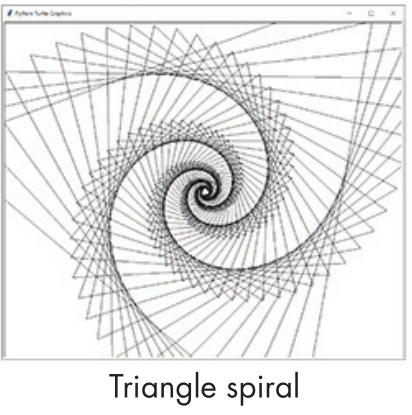The Triangle Spiral fractal, another variation of the Spiral Square, uses the `drawTriangleOutline()` shape-drawing function instead of `drawFilledSquare()`:

```# Triangle Spiral:
drawFractal(drawTriangleOutline, 20, [{'sizeChange': 1.05, 'angleChange': 7}], 80)
```

Unlike the Spiral Square, the Triangle Spiral begins at the small size of `20` units and slightly increases in size for each level of recursion. The `sizeChange` key is greater than `1.0`, so the shapes are always increasing in size.

This means the base case occurs when the recursion reaches a depth of `80`, because the base case of size becoming less than `1` is never reached.

## Example 5 - Conway's Game of Life Glider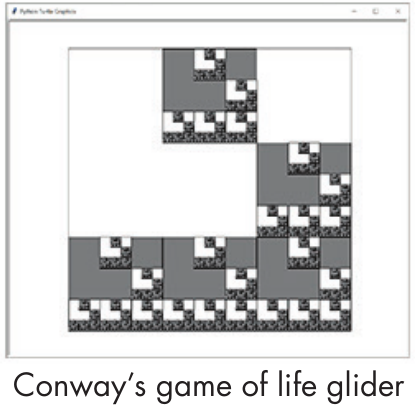Conway's Game of Life is a famous example of cellular automata. The game's simple rules cause interesting and wildly chaotic patterns to emerge on a two-dimensional grid. One such pattern is a Glider consisting of five cells in a 3 × 3 space:

```# Conway's Game of Life Glider:
third = 1 / 3
drawFractal(drawFilledSquare, 600,
[{'sizeChange': third, 'yChange': third},
{'sizeChange': third, 'xChange': third},
{'sizeChange': third, 'xChange': third, 'yChange': -third},
{'sizeChange': third, 'yChange': -third},
{'sizeChange': third, 'xChange': -third, 'yChange': -third}])
```

The Glider fractal here has additional Gliders drawn inside each of its five cells. The third variable helps precisely set the position of the recursive shapes in the 3 × 3 space.

You can find a Python implementation of Conway's Game of Life online at https://inventwithpython.com/bigbookpython/project13.html. Tragically, John Conway, the mathematician and professor who developed Conway's Game of Life, passed away of complications from COVID-19 in April 2020.

## Example 6 - Sierpiński TriangleWe created the Sierpiński Triangle fractal in Chapter 9, but our Fractal Art Maker can re-create it as well by using the `drawTriangleOutline()` shape function. After all, a Sierpiński triangle is an equilateral triangle with three smaller equilateral triangles drawn in its interior:

```# Sierpinski Triangle:
toMid = math.sqrt(3) / 6
drawFractal(drawTriangleOutline, 600,
[{'sizeChange': 0.5, 'yChange': toMid, 'angleChange': 0},
{'sizeChange': 0.5, 'yChange': toMid, 'angleChange': 120},
{'sizeChange': 0.5, 'yChange': toMid, 'angleChange': 240}])
```

The center of these smaller triangles is `size * math.sqrt(3) / 6` units from the center of the previous triangle. The three calls adjust the heading of the turtle to 0, 120, and 240 degrees before moving up on the turtle's relative y-axis.

## Example 7 - WaveWe discussed the Wave fractal at the start of this chapter. This relatively simple fractal creates three smaller and distinct recursive triangles:

```# Wave:
drawFractal(drawTriangleOutline, 280,
[{'sizeChange': 0.5, 'xChange': -0.5, 'yChange': 0.5},
{'sizeChange': 0.3, 'xChange': 0.5, 'yChange': 0.5},
{'sizeChange': 0.5, 'yChange': -0.7, 'angleChange': 15}])
```

## Example 8 - Horn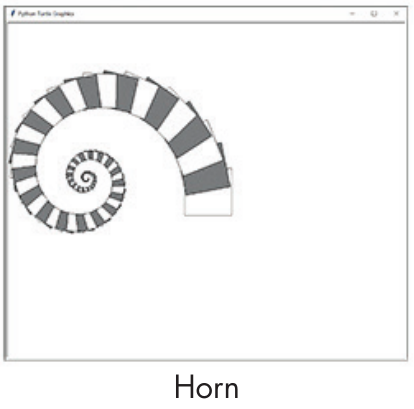The Horn fractal resembles a ram's horn:

```# Horn:
drawFractal(drawFilledSquare, 100, [{'sizeChange': 0.96, 'yChange': 0.5, 'angleChange': 11}], 100)
```

This simple fractal is made up of squares, each of which is slightly smaller, moved up, and rotated 11 degrees from the previous square. We increase the maximum recursion depth to 100 to extend the horn into a tight spiral.

## Example 9 - SnowflakeThe final fractal, Snowflake, is composed of squares laid out in a pentagon pattern. This is similar to the Four Corners fractal, but it uses five evenly spaced recursive squares instead of four:

```# Snowflake:
drawFractal(drawFilledSquare, 200,
[{'xChange': math.cos(0 * math.pi / 180),
'yChange': math.sin(0 * math.pi / 180), 'sizeChange': 0.4},
{'xChange': math.cos(72 * math.pi / 180),
'yChange': math.sin(72 * math.pi / 180), 'sizeChange': 0.4},
{'xChange': math.cos(144 * math.pi / 180),
'yChange': math.sin(144 * math.pi / 180), 'sizeChange': 0.4},
{'xChange': math.cos(216 * math.pi / 180),
'yChange': math.sin(216 * math.pi / 180), 'sizeChange': 0.4},
{'xChange': math.cos(288 * math.pi / 180),
'yChange': math.sin(288 * math.pi / 180), 'sizeChange': 0.4}])
```

This fractal uses the cosine and sine functions from trigonometry, implemented in Python's `math.cos()` and `math.sin()` functions, to determine how to shift the squares along the x-axis and y-axis. A full circle has 360 degrees, so to evenly space out the five recursive squares in this circle, we place them at intervals of 0, 72, 144, 216, and 288 degrees. The `math.cos()` and `math.sin()` functions expect the angle argument to be in radians instead of degrees, so we must multiply these numbers by `math.pi / 180.`

The end result is that each square is surrounded by five other squares, which are surrounded by five other squares, and so on, to form a crystal-like fractal that resembles a snowflake.